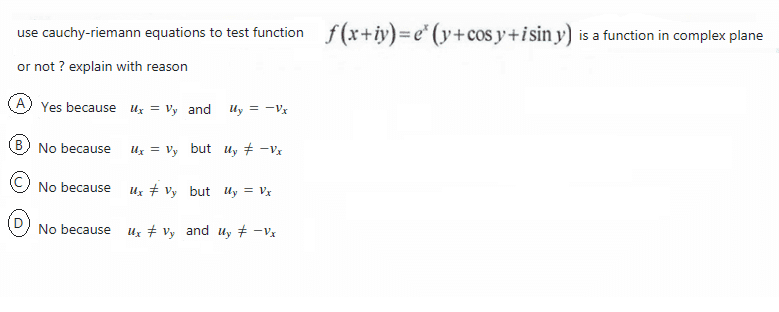# Cauchy-Riemann Equations test problem

• MHB
• jaychay
In summary, Klaas stated that $u = \mathcal{Re}\left( f \right) = \mathrm{e}^x \left[ y + \cos{ \left( y \right) } \right]$ and $v = \mathcal{Im}\left( f \right) = \mathrm{e}^x \sin{ \left( y \right) }$.f

#### jaychayI cannot figure it out which choice is correct

What is the real part of $f(x+iy)$? That is $u(x,y)$.
What is its partial derivative with respect to $x$? That is $u_x$.

What is the real part of $f(x+iy)$? That is $u(x,y)$.
What is its partial derivative with respect to $x$? That is $u_x$.
Is the correct answer is choice D ?
I already done it by using partial derivatives and compare them

Is the correct answer is choice D ?
I already done it by using partial derivatives and compare them
It seems you are doing some online exam.
I'd rather not validate answers for something like that.

It seems you are doing some online exam.
I'd rather not validate answers for something like that.
Sir, it's not an online exam
It's my assignment that I try to do on my own
I translate it from my math textbook in my country into English
I really want to become better at math.

If you still don't believe or you think I try to lie to you
Here is the original form of math textbook in my country#### Attachments

Have you at least established what your u and v are?

As Klaas stated, \displaystyle \begin{align*} u = \mathcal{Re}\left( f \right) = \mathrm{e}^x \left[ y + \cos{ \left( y \right) } \right] \end{align*} and \displaystyle \begin{align*} v = \mathcal{Im}\left( f \right) = \mathrm{e}^x \sin{ \left( y \right) } \end{align*}. Surely you can evaluate the necessary partial derivatives and make a judgement...

Have you at least established what your u and v are?

As Klaas stated, \displaystyle \begin{align*} u = \mathcal{Re}\left( f \right) = \mathrm{e}^x \left[ y + \cos{ \left( y \right) } \right] \end{align*} and \displaystyle \begin{align*} v = \mathcal{Im}\left( f \right) = \mathrm{e}^x \sin{ \left( y \right) } \end{align*}. Surely you can evaluate the necessary partial derivatives and make a judgement...
Thank you very much for guiding it for me.